# Quasi-analytic class

(diff) ← Older revision | Latest revision (diff) | Newer revision → (diff)

of functions

A class of functions characterized by a uniqueness property: If two functions of the class coincide "locally" , then they are identical. The simplest quasi-analytic class is the class of analytic functions on an intervalon the real axis (a function of this class is represented in a sufficiently small neighbourhood of each point of the interval by a Taylor series): If two analytic functions onare equal on an interval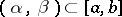, then they are identical (to coincide "locally" here means equality of the functions in the interior of). Coincidence "locally" for analytic functions can also mean equality of the functions together with all their derivatives at some point,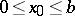. Coincidence "locally" in this new sense also implies equality of the functions on the whole interval.

E. Borel discovered that the uniqueness property holds not merely for analytic functions. In this connection J. Hadamard, 1912, posed the following problem. Let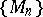be a sequence of positive numbers and letbe some interval on the real axis. Let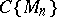be the set of infinitely-differentiable functionsonfor which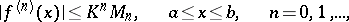where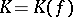is a constant not dependent on. A functionis analytic onif and only if for some,(1)

Thus, the class of analytic functions onis the class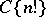. Hadamard's problem consists in determining conditions on the numbers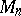such that every function in the classvanishing together with each of its derivatives at some point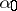,, is identically zero (or, what is the same, such that two functions inthat are equal together with all their derivatives at a pointare equal everywhere). A classwith this property is called quasi-analytic on. According to what has been said above, the classis quasi-analytic on.

A. Denjoy, 1921, gave sufficient conditions for quasi-analyticity. He pointed out that if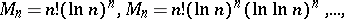thenis quasi-analytic (these classes are, by virtue of (1), wider than the class of analytic functions).

T. Carleman completely solved Hadamard's problem by giving necessary and sufficient conditions for quasi-analyticity. These conditions were subsequently modified. The Denjoy–Carleman quasi-analyticity theorem is stated as follows: Each of the following conditions is necessary and sufficient for the quasi-analyticity of the class:

a) if one setsthen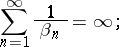b) if one sets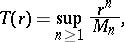thenc) eitheror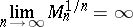andwhere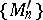is the convex regularization by means of logarithms of the sequence. Condition a) is called the Carleman condition, b) the Ostrowski condition, c) the Wang–Mandelbrojt condition.

For the case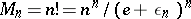(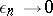as), one has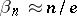, condition a) holds, and again one finds thatis a quasi-analytic class. For the case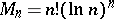, one has, condition a) holds, and hence the Denjoy class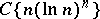is quasi-analytic. In the case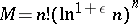,, one hasas a result of whichis not quasi-analytic.

S.N. Bernshtein introduced other quasi-analytic classes of functions. He showed that a functionis analytic on an intervalif and only if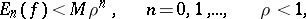whereand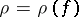do not depend on, andis the best approximation error ofonby polynomials of degree. With this in mind, he considered the class of functionsonsatisfying the condition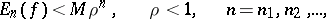(2)

where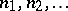is an infinite increasing sequence of integers, and showed that if a function of this class vanishes on some interval, then it is identically zero. A classdefined onis called (Bernshtein) quasi-analytic if two functions of this class that are equal on some segmentare necessarily equal on the whole of. The class (2) is quasi-analytic in this sense. It should be noted that (2) does not imply thatis infinitely differentiable (there are appropriate examples).

Other problems of quasi-analyticity have also been studied. For example, the question has been solved concerning the rate of decrease of the coefficients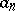andin the series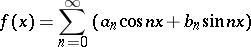under which the class of such functions is quasi-analytic; conditions have been found on the numberssuch that functionsthat are analytic in the disc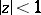, are infinitely differentiable on the closed disc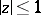and satisfy the conditionsform a quasi-analytic class; etc.

How to Cite This Entry:
Quasi-analytic class. Encyclopedia of Mathematics. URL: http://encyclopediaofmath.org/index.php?title=Quasi-analytic_class&oldid=11432
This article was adapted from an original article by A.F. Leont'ev (originator), which appeared in Encyclopedia of Mathematics - ISBN 1402006098. See original article